# Fractions

How many equal parts of a whole

### Slice a pizza, and we get fractions:1/2 1/4 3/8 (One-Half) (One-Quarter) (Three-Eighths)

The top number says how many slices we have.
The bottom number says how many equal slices the whole pizza was cut into.

## Equivalent Fractions

Some fractions may look different, but are really the same, for example:

 4/8 = 2/4 = 1/2 (Four-Eighths) (Two-Quarters) (One-Half)=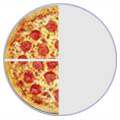=It is usually best to show an answer using the simplest fraction ( 1/2 in this case ). That is called Simplifying, or Reducing the Fraction

## Numerator / Denominator

We call the top number the Numerator, it is the number of parts we have.
We call the bottom number the Denominator, it is the number of parts the whole is divided into.

NumeratorDenominator

You just have to remember those names! (If you forget just think "Down"-ominator)

It is easy to add fractions with the same denominator (same bottom number):

 1/4 + 1/4 = 2/4 = 1/2 (One-Quarter) (One-Quarter) (Two-Quarters) (One-Half)+==One-quarter plus one-quarter equals two-quarters, equals one-half

Another example:

 5/8 + 1/8 = 6/8 = 3/4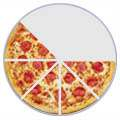+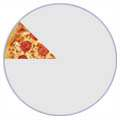=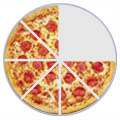=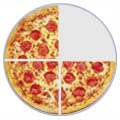Five-eighths plus one-eighth equals six-eighths, equals three-quarters

## Adding Fractions with Different Denominators

But what about when the denominators (the bottom numbers) are not the same?

 3/8 + 1/4 = ?+=Three-eighths plus one-quarter equals ... what?

We must somehow make the denominators the same.

In this case it is easy, because we know that 1/4 is the same as 2/8 :

 3/8 + 2/8 = 5/8+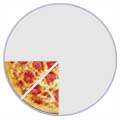=Three-eighths plus two-eighths equals five-eighths

There are two popular methods to make the denominators the same:

(They both work nicely, use the one you prefer.)

## Other Things We Can Do With Fractions

We can also:

Visit the Fractions Index to find out even more.